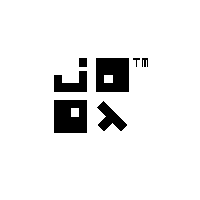{{announcement.body}}
{{announcement.title}}

# Java 8 Functional Programming with jOOλ Example

DZone 's Guide to

# Java 8 Functional Programming with jOOλ Example

### The Java 8 Stream API does not offer enough functionality for this task, so this quick tutorial will use jOOλ.

· Java Zone ·
Free Resource

Comment (0)

Save
{{ articles.views | formatCount}} Views

I’ve stumbled upon an interesting Stack Overflow question by user “mip”. The question was:

I’m looking for a way of generating an alphabetic sequence:

``A, B, C, ..., Z, AA, AB, AC, ..., ZZ.``

This can be quickly recognized as the headings of an Excel spreadsheet, which does precisely that:So far, none of the answers employed any Java 8 functional programming, which I accepted as a challenge. We’re going to use jOOλ, because the Java 8 Stream API does not offer enough functionality for this task.

But first, let’s decompose the algorithm in a functional way. What we need are these components:

1. A (reproducible) representation of the alphabet
2. An upper bound, i.e. how many letters we want to produce. The requested sequence goes to `ZZ`, which means the upper bound would be 2
3. A way to combine each letter of the alphabet with the previously generated combined letters in a cartesian product

Let’s look into some code:

### 1. Generating the Alphabet

We could be writing the alphabet like this:

``List<String> alphabet = Arrays.asList("A", "B", ..., "Z");``

but that would be lame. Let’s generate it instead, using jOOλ:

``````List<String> alphabet = Seq
.rangeClosed('A', 'Z')
.map(Object::toString)
.toList();``````

The above generates a “closed” range (Java-8-Stream-speak for a range with inclusive upper bound) of characters between `A` and `Z`, maps characters to strings and collects them into a list.

So far so good. Now:

### 2. Using an Upper Bound

The requested sequence of characters includes:

``A .. Z, AA, AB, .. ZZ``

But we could easily imagine extending this requirement generally to produce the following, or even more.

``A .. Z, AA, AB, .. ZZ, AAA, AAB, .. ZZZ``

For this, we’ll use `rangeClosed()` again:

``````// 1 = A .. Z, 2 = AA .. ZZ, 3 = AAA .. ZZZ
Seq.rangeClosed(1, 2)
.flatMap(length -> ...)
.forEach(System.out::println);``````

The idea here is to produce a new stream for each individual length in the range `[1 .. 2]`, and to flatten those streams into one single stream. `flatMap()` is essentially the same as a nested loop in imperative programming.

### 3. Combine Letters in a Cartesian Product

This is the trickiest part: We need to combine each letter with each letter `length` times. For this, we’ll use the following stream:

``````Seq.rangeClosed(1, length - 1)
.foldLeft(Seq.seq(alphabet), (s, i) ->
s.crossJoin(Seq.seq(alphabet))
.map(t -> t.v1 + t.v2))
);``````

We’re using again `rangeClosed()` to produce values in the range `[1 .. length-1]`. `foldLeft()` is the same as `reduce()`, except that `foldLeft()` is guaranteed to go from “left to right” in a stream, without requiring the folding function to be associative. Whew.

In other, more understandable terms: `foldLeft()` is nothing else but an imperative loop. The “seed” of the loop, i.e. the loop’s initial value, is a complete alphabet (`Seq.seq(alphabet)`). Now, for every value in the range `[1 .. length-1]`, we produce a cartesian product (`crossJoin()`) between the letters “folded” so far and a new alphabet, and we concatenate each combination into a single new string (`t.v1` and `t.v2`).

That’s it!

### Combining Everything

The following simple program prints all the values from `A .. Z, AA .. ZZ, AAA .. ZZZ` to the console:

``````import java.util.List;

import org.jooq.lambda.Seq;

public class Test {
public static void main(String[] args) {
int max = 3;

List<String> alphabet = Seq
.rangeClosed('A', 'Z')
.map(Object::toString)
.toList();

Seq.rangeClosed(1, max)
.flatMap(length ->
Seq.rangeClosed(1, length - 1)
.foldLeft(Seq.seq(alphabet), (s, i) ->
s.crossJoin(Seq.seq(alphabet))
.map(t -> t.v1 + t.v2)))
.forEach(System.out::println);
}
}``````

### Disclaimer

This is certainly not the most optimal algorithm for this particular case. One of the best implementations has been given by an unnamed user on Stack Overflow:

``````import static java.lang.Math.*;

private static String getString(int n) {
char[] buf = new char[(int) floor(log(25 * (n + 1)) / log(26))];
for (int i = buf.length - 1; i >= 0; i--) {
n--;
buf[i] = (char) ('A' + n % 26);
n /= 26;
}
return new String(buf);
}``````

Needless to say that the latter runs much much faster than the previous functional algorithm.

Topics:
functional programming ,java 8

Comment (0)

Save
{{ articles.views | formatCount}} Views

Published at DZone with permission of Lukas Eder , DZone MVB. See the original article here.

Opinions expressed by DZone contributors are their own.